【機器學習演算法實現】系列文章將記錄個人閱讀機器學習論文、書籍過程中所碰到的演算法，每篇文章描述一個具體的演算法、演算法的程式設計實現、演算法的具體應用例項。爭取每個演算法都用多種語言程式設計實現。所有程式碼共享至github：https://github.com/wepe/MachineLearning-Demo     歡迎交流指正！

（2）logistic迴歸__基於Python和Numpy函式庫

1、演算法簡介

logistic迴歸演算法之所以稱作“logistic”，是因為它運用了logistic函式，即sigmoid函式

logistic迴歸演算法一般用於二分類問題（當然也可以多類別，後面會講）。

logistic迴歸的演算法思想：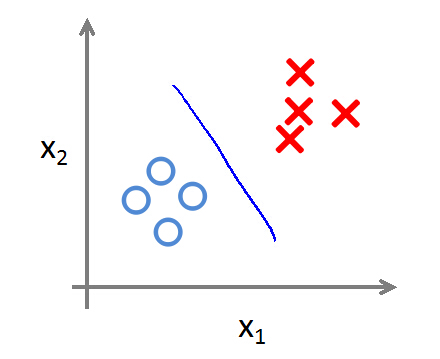logistic迴歸的最後一步就是將f(x)作為輸入，代入Sigmoid函式，當f(x)>0時，sigmoid函式的輸出就大0.5，且隨

f(x)趨於正無窮，sigmoid函式的輸出趨於1。當f(x)<0時，sigmoid函式的輸出就小於0.5，且隨f(x)趨於負無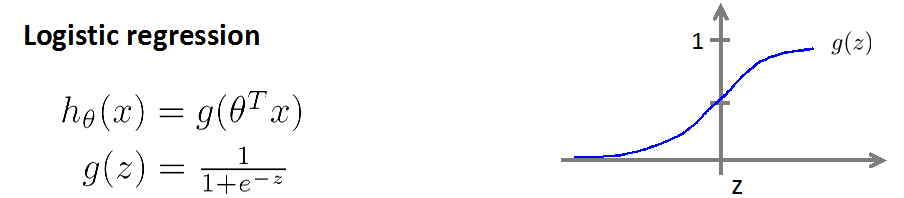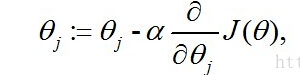alpha是步長，一系列推導後：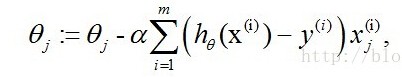2、工程例項

logistic迴歸一般用於二分類問題，比如判斷一封郵件是否為垃圾郵件，判斷照片中的人是男是女，預測一場比賽輸還

1作為一個類別，0、2～9作為一個類別，這樣就可以識別出1或非1........

（1）工程檔案說明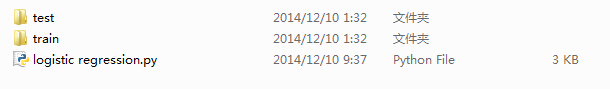logistic regression.py實現的功能：從train裡面讀取訓練資料，然後用梯度上升演算法訓練出引數Θ，接著用引數Θ來預

test裡面的測試樣本，同時計算錯誤率。

（2）原始碼解釋

def loadData(direction):    trainfileList=listdir(direction)    m=len(trainfileList)    dataArray= zeros((m,1024))    labelArray= zeros((m,1))    for i in range(m):        returnArray=zeros((1,1024))  #每個txt檔案形成的特徵向量        filename=trainfileList[i]        fr=open('%s/%s' %(direction,filename))        for j in range(32):            lineStr=fr.readline()            for k in range(32):                returnArray[0,32*j+k]=int(lineStr[k])        dataArray[i,:]=returnArray   #儲存特徵向量            filename0=filename.split('.')        label=filename0.split('_')        labelArray[i]=int(label)     #儲存類別    return dataArray,labelArray

• sigmoid(inX)函式
def sigmoid(inX):    return 1.0/(1+exp(-inX))

def gradAscent(dataArray,labelArray,alpha,maxCycles):    dataMat=mat(dataArray)    #size:m*n    labelMat=mat(labelArray)      #size:m*1    m,n=shape(dataMat)    weigh=ones((n,1))     for i in range(maxCycles):        h=sigmoid(dataMat*weigh)        error=labelMat-h    #size:m*1        weigh=weigh+alpha*dataMat.transpose()*error    return weigh

• classfy(testdir,weigh)函式

def classfy(testdir,weigh):    dataArray,labelArray=loadData(testdir)    dataMat=mat(dataArray)    labelMat=mat(labelArray)    h=sigmoid(dataMat*weigh)  #size:m*1    m=len(h)    error=0.0    for i in range(m):        if int(h[i])>0.5:            print int(labelMat[i]),'is classfied as: 1'            if int(labelMat[i])!=1:                error+=1                print 'error'        else:            print int(labelMat[i]),'is classfied as: 0'            if int(labelMat[i])!=0:                error+=1                print 'error'    print 'error rate is:','%.4f' %(error/m)

• digitRecognition(trainDir,testDir,alpha=0.07,maxCycles=10)函式

3、試驗結果

>>> digitRecognition('train','test')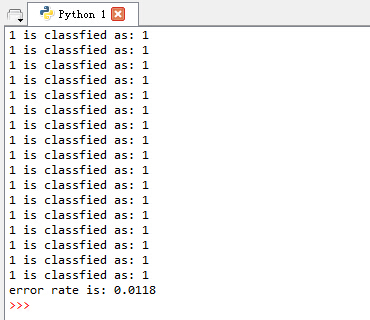>>> digitRecognition('train','test',0.01,50)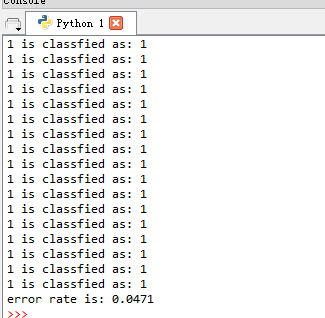給我老師的人工智慧教程打call！http://blog.csdn.net/jiangjunshow